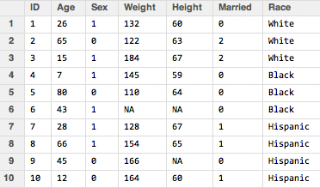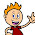## Friday, November 30, 2012

### Data types part 4: Logical class

First, an update:  A commentator has asked me to post my code so that it is easier to practice the examples I show here.  It will take me a little bit of time to get all of my code for past posts well-documented and readable, but I have uploaded the code and data for the last 4 posts, including this one, here:

Unfortunately, I could not find a way to attach it to blogger, so sorry for the extra step.
_________________________________________________________________________

Ok, now on to Data types part 4: Logical

I started this series of posts on data types by saying that when you have a dataframe like this called mydata:you can't do this in R:

Age<25

Because Age does not exist as an object in R, and you get the error below:

But then what happens when I do,

mydata\$Age<25

This is perfectly legal to do in R, but it's not going to drop observations. With this kind of statement, you are asking R to evaluate the logical question "Is it true that mydata\$Age is less than 25?".  Well, that depends on which element of the Age vector, of course. Which is why this is what you get when you run that code:On first glance, this looks like a character vector.  There is a string of entries using character letters after all.  But it's not character class, it's the logical class.  If you save this string of TRUE and FALSE entries into an object and print its class, this is what you get:The logical class can only take on two values, TRUE or FALSE.  We've seen evaluations of logical operations already, first in subsetting, like this:

mysubset<-mydata[mydata\$Age<40,]

Check out my post on subsetting if this syntax is confusing. In a nutshell, R evaluates all rows and keeps only those that meet the criteria, which is only rows where Age has a value of under 40 and all columns.

Or here, in ifelse() statements

mydata\$Young<-ifelse(mydata\$Age<25,1,0)

More on ifelse() statements here. The ifelse() function is really useful, but is actually overkill when you're just creating a binary variable. This can be done faster by taking advantage of the fact that logical values of TRUE always have a numeric value of 1, while logical values of FALSE always have a numeric value of 0.

That means all I need to do to create a binary variable of under age 25 is to convert my logical mydata\$Ageunder25 vector into numeric.  This is very easy with R's as.numeric() function. I do it like this:

mydata\$Ageunder25_num<-as.numeric(mydata\$Ageunder25)

or directly without that intermediate step like this:

mydata\$Ageunder25_num<-as.numeric(mydata\$Age<25)

Let's check out the relevant columns in our dataframe:

We can see that the Ageunder25_num variable is an indicator of whether the Age variable is under 25.

Now the really, really useful part of this is that you can use this feature to turn on and off a variable depending on its value. For example, say you got your data and realized that some of the height values were in inches and some were in centimeters, like this:Those heights of 152 and 170 are in centimeters while everything else is inches.  There are various ways to fix it, but one way is to check which values are less than, say 90, which is probably a safe cutoff and create a new column that keeps those values under 90 but converts the values over 90.  We can do this in this way:

mydata\$Height_fixed_in<-  as.numeric(mydata\$Height_wrong<90)*mydata\$Height_wrong
+ as.numeric(mydata\$Height_wrong>=90)*mydata\$Height_wrong/2.54

So the first half of the calculation (in red) is "turned on" when Height_wrong is less than 90, because the value of the logical statement is a numeric TRUE, i.e. a 1, and this value of 1 is multiplied by the original Height column.  The second part of the statement (in blue) is FALSE and so is just 0 times something so it's 0.  If the Height_wrong column is greater than 90, then the first half is just 0 and the second half  is turned on and thus the Height_wrong variable is divided by 2.54 cm, converting it into inches. We get the result below:

Another useful way to use the as.numeric() and logical classes to your advantage is a situation like this:

I have in my dataset the age of the last child born (and probably other characteristics of this child not shown), and then just the number of other children for each woman.  I want to get a total number of children variable.  I can do it simply in the following way.

First, a note about the is.na() function.  If you want to check if a variable is missing in R, you don't use syntax like "if variable==NA" or "if variable==.".  This is not going to indicate a missing value. What you want to use instead is is.na(variable) like this:

is.na(newdata\$Child1age)

Which gives you a logical vector that looks like this:

If you want to check if a variable is not missing, you use the ! sign (meaning "Not") in front and check it like this:

We've seen this kind of thing before!  Now we can translate this logical vector into numeric and add it to the number of other children, like this:

newdata\$Totalnumchildren<-as.numeric(!is.na(newdata\$Child1age))+newdata\$Numotherchildren

We get the following:

If we want to get those NAs to be 0, we can again use the is.na() function and replace whereever Totalnumchildren is missing with a 0 like this:

newdata\$Totalnumchildren[is.na(newdata\$Totalnumchildren)]<-0

1.These tricks are lovely!

1.Thank you! :)

2.Nice tricks. Its hard to get a Health Data Management Services.

Note: Only a member of this blog may post a comment.